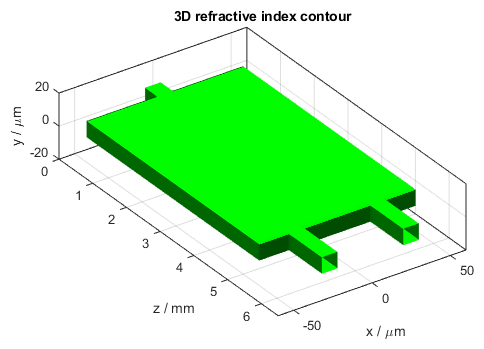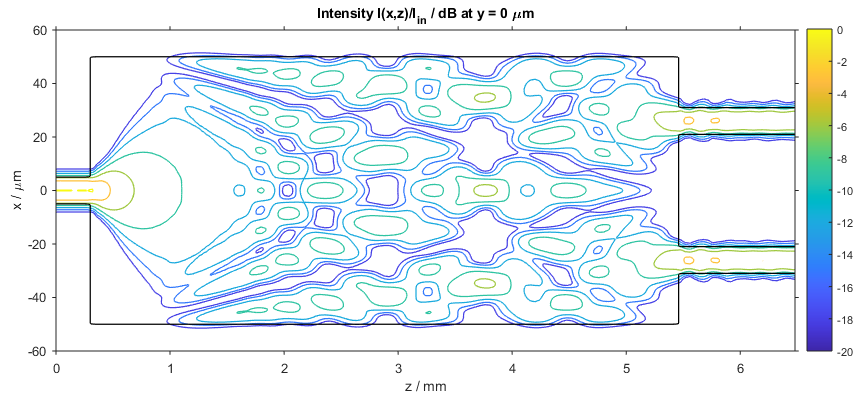# Multimode Interference Coupler

This BeamLab demo shows how a multimode interference (MMI) coupler uniformly distributes the light of a single-mode input waveguide to two single-mode output waveguides. First, the fundamental mode of the input single-mode waveguide is calculated and used as input for the beam propagation simulation. During the beam propagation the fundamental mode then spreads out inside the multimode waveguide and interferes with its reflections from the waveguide boundaries. At a certain distance, the interference pattern is such that the multimode waveguide efficiently couples light into the two output waveguides.

BeamLab demo: mmi_coupler_3d.m

The waveguide structure consists of a square single-mode waveguide in the input section, a rectangular multi-mode waveguide in the interference section, and two square single-mode waveguides in the output section. The length of the interference section is chosen such that the light is most efficiently coupled into the two output waveguides.3D refractive index contour of the MMI coupler

The following video shows how the intensity distribution changes along the propagation distance z. The left figure shows the two-dimensional intensity distribution I(x,y) in form of intensity contour lines and the right figure shows the one-dimensional intensity distribution I(x) at y = 0 µm. The black lines indicate the location of the waveguide.

Video of 2D (left) and 1D (right) intensity profiles

After the calculation has finished, the intensity distribution as a function of propagation distance z is plotted. The following figure shows the intensity distribution I(x,z) at y = 0 µm in form of intensity contour lines.Intensity distribution in the MMI coupler (xz slice)